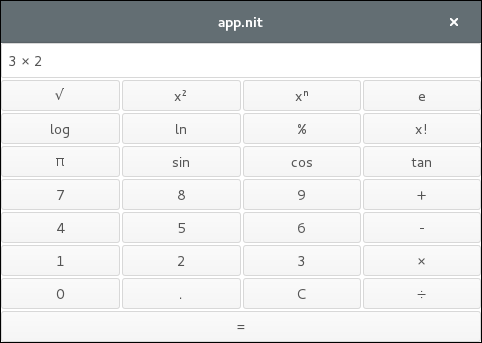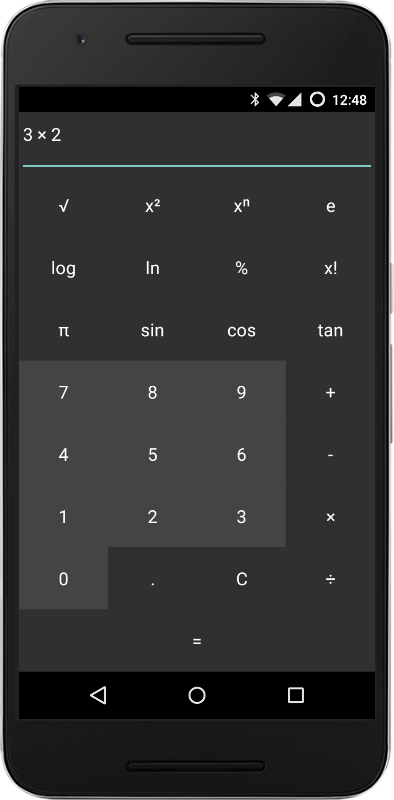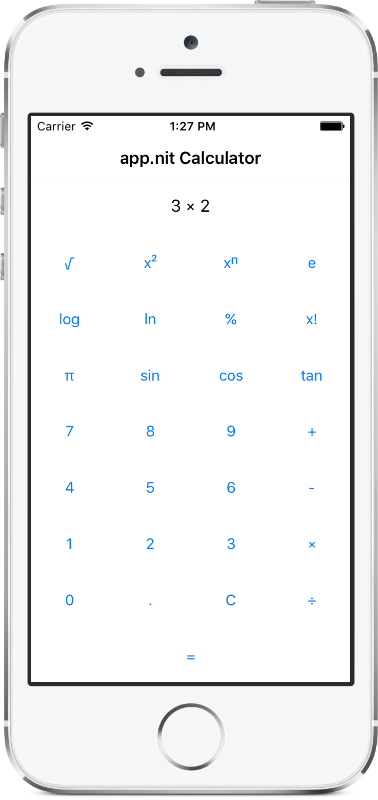# Project structure

• `calculator_logic` defines `CalculatorContext` with all the business logic of a calculator. It takes as input operations and numbers, and outputs the text to display.
• `calculator` implements the portable graphical interface using the app.nit framework
• `scientific_calculator` refines `calculator` to add scientific operations.
• `android_calculator` refines `calculator` to get a nicer aesthetic on Android.
• `ios_calculator` refines `calculator` to get a nicer aesthetic on iOS.
• `calculator_test` test `CalculatorContext` as a black box.

# Compilation

• Compile and run on the desktop (GNU/Linux and OS X) with:

``````bin/calculator
``````
• Compile for Android and install on a device or emulator with:

``````make bin/android.apk
``````
• Compile for iOS and run on the simulator with:

``````make bin/android.app
ios-sim launch bin/calculator.app
``````

# Screenshots## Content

• calculator: Portable calculator built using app.nit (examples/calculator)
• android (examples/calculator/android)
• res (examples/calculator/android/res)
• src (examples/calculator/src)
• android14: Aesthetic variation for Android for API 14+ (examples/calculator/src/android14.nit)
• android21 (examples/calculator/src/android21)
• android (examples/calculator/src/android21/android)
• res (examples/calculator/src/android21/android/res)
• android21: Aesthetic variation for Android Lollypop (API 21) (examples/calculator/src/android21/android21.nit)
• calculator: Portable calculator UI (examples/calculator/src/calculator.nit)
• calculator_logic: Business logic of a calculator (examples/calculator/src/calculator_logic.nit)
• calculator_test: Test the business logic (examples/calculator/src/calculator_test.nit)
• ios: Aesthetic variation for iOS (examples/calculator/src/ios.nit)
• scientific (examples/calculator/src/scientific)
• scientific: Extends the portable calculator app with scientific operations (examples/calculator/src/scientific/scientific.nit)